• Save

Embedded Figure Test - Non Verbal Reasoning Questions and Answers

4 months ago 5.2K Views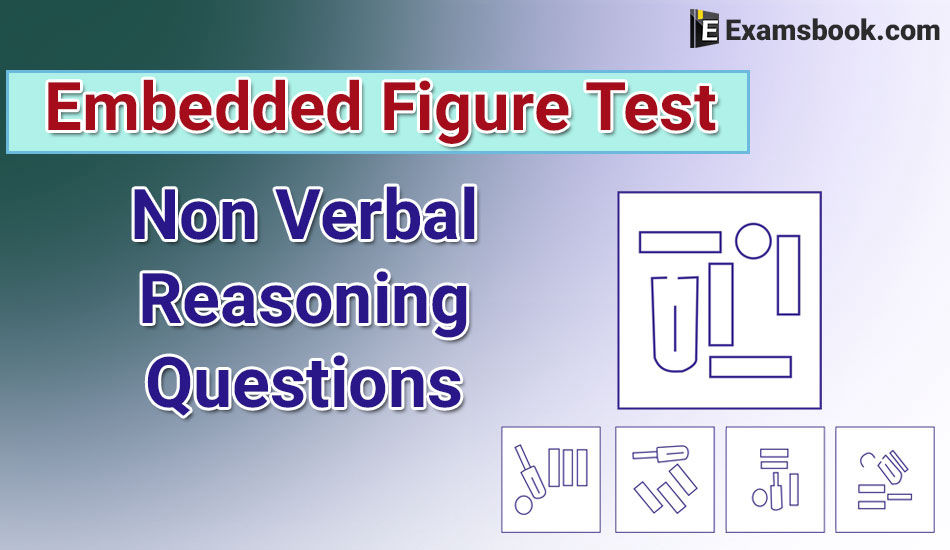Most of the students ignore Embedded Figure Test questions and answers. But embedded figure test non-verbal reasoning questions are very important for competitive exams. So, students should practice these embedded figure test - non verbal reasoning questions and answers to get better scoring.

Here are the important and selective embedded figure test non-verbal questions with answers which are helpful for students to cover all competitive exams and you can easily get better rank with these embedded figure test questions and increase your performance level.

To improve your performance you can practice more with Embedded Figures Test Online Free Questions.

Embedded Figure Test Non Verbal Reasoning Questions and Answers:

Q.1. Which figure includes all the specific parts of the question figure?

Question figureAnswer figure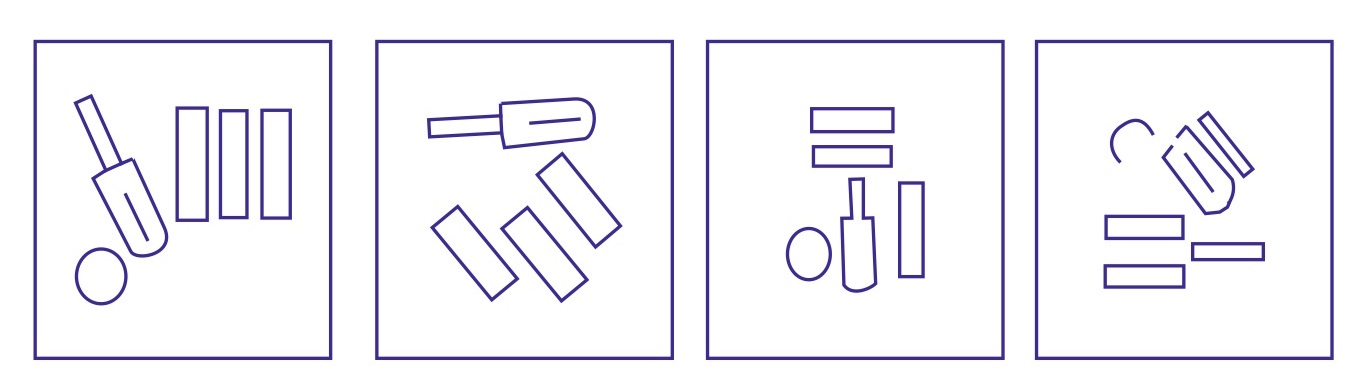(A)                                   (B)                               (C)                       (D)

Ans .   A

Q.2. Find the figure which includes the question figure.

Question figure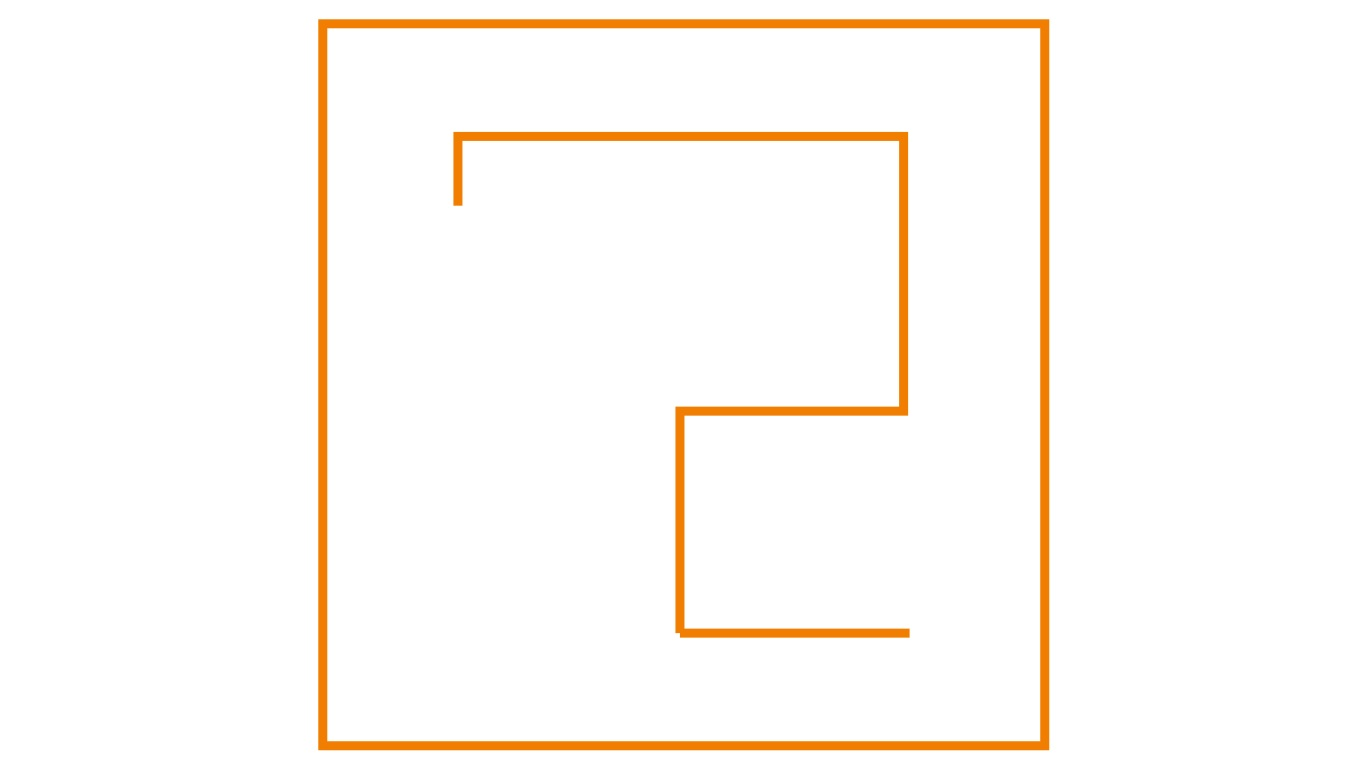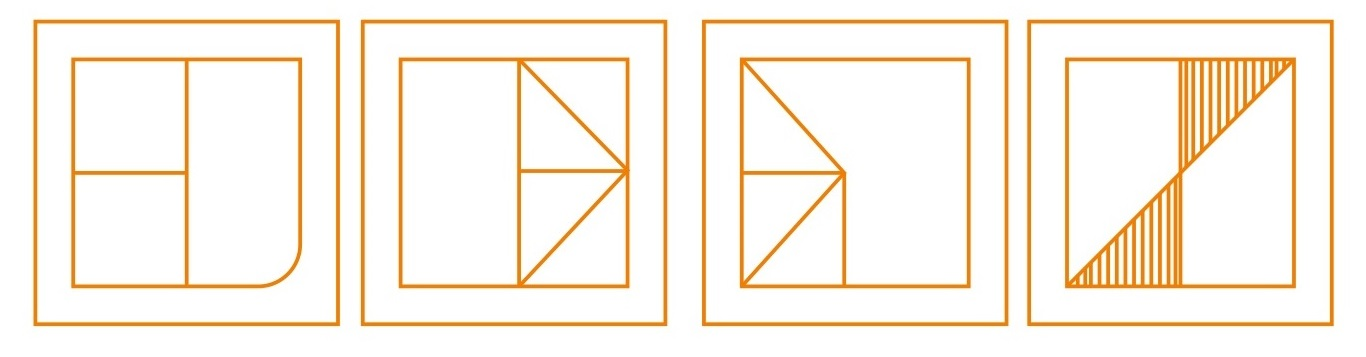(A)                                       (B)                                (C)                          (D)

Ans .   B

Q.3. Which of the answer figure in the following includes the question figure?

Question figure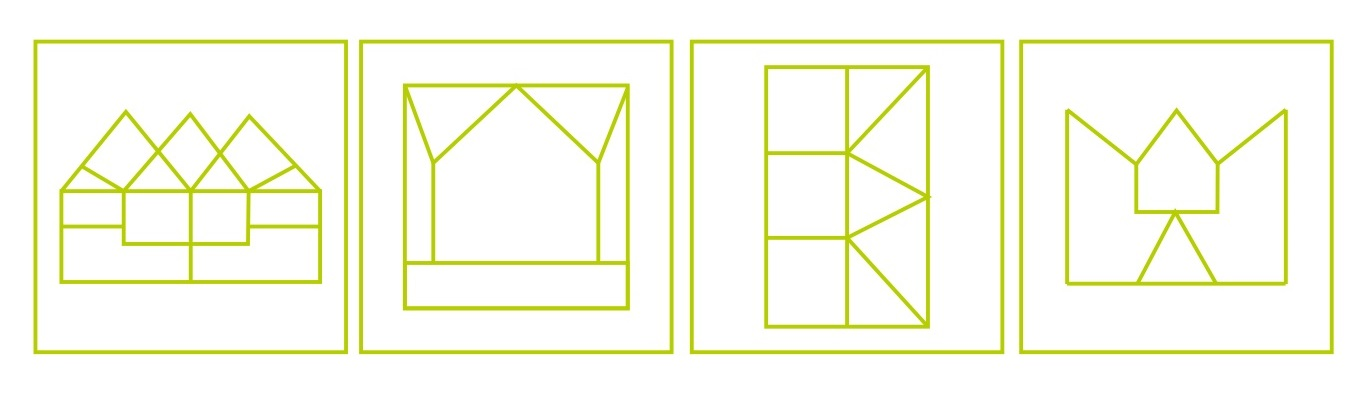(A)                              (B)                              (C)                      (D)

Ans .   A

Q.4.Find the answer figure which includes the question figure.

Question figure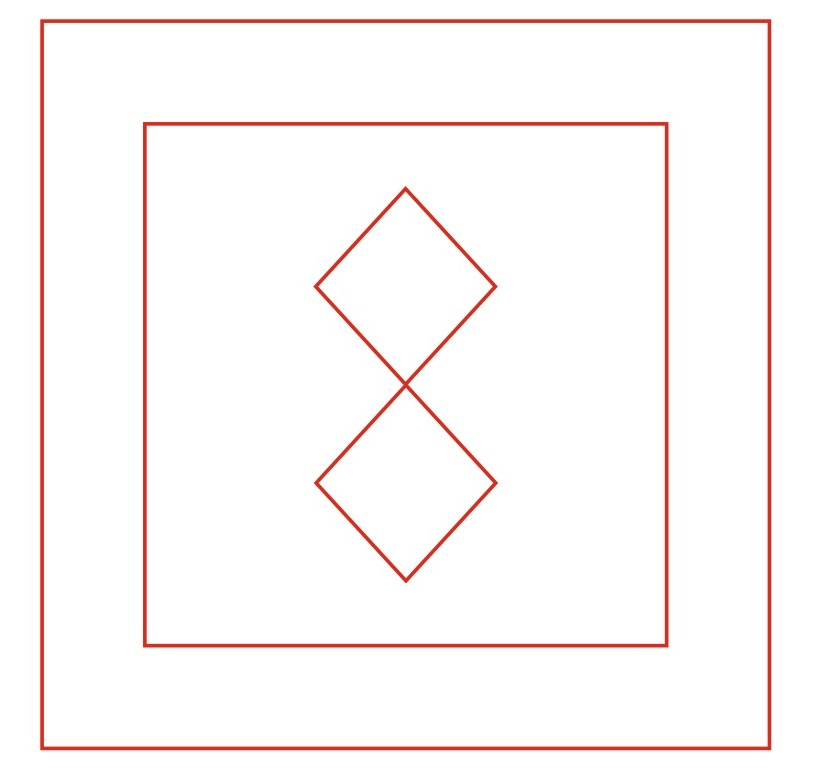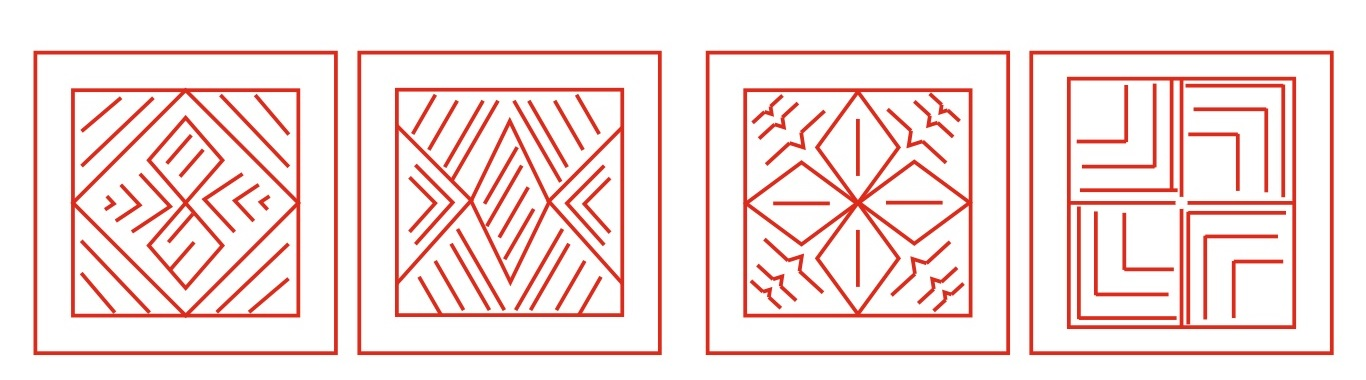(A)                                   (B)                                (C)                               (D)

Ans .   A

Q.5. Find the answer figure which includes the question figure.

Question figure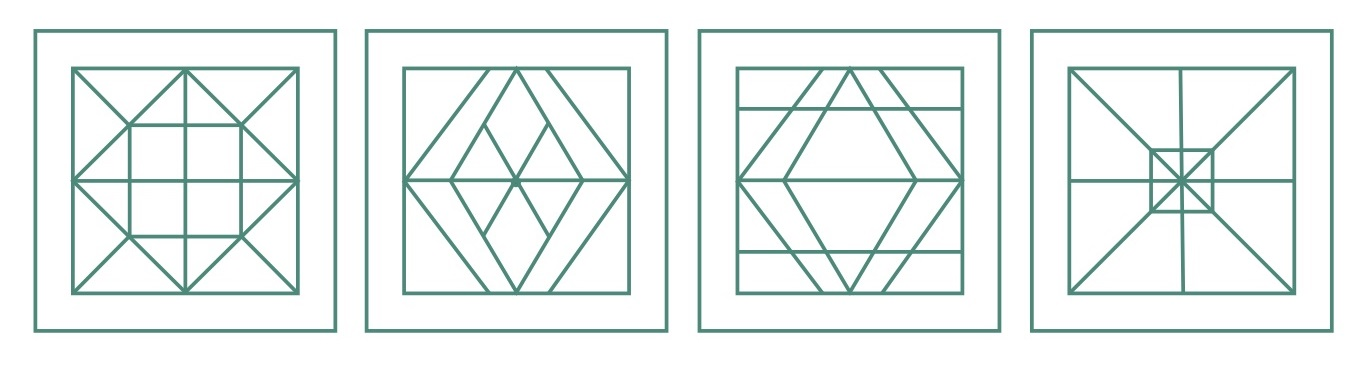(A)                                 (B)                           (C)                           (D)

Ans .   C

Q.6. In which answer figure, the question figure is included?

Question figure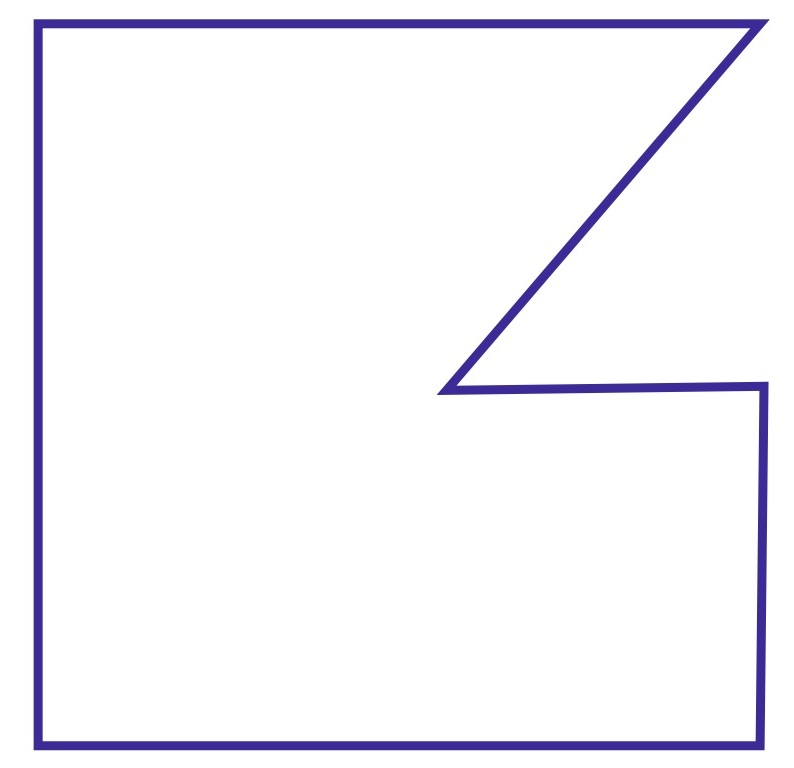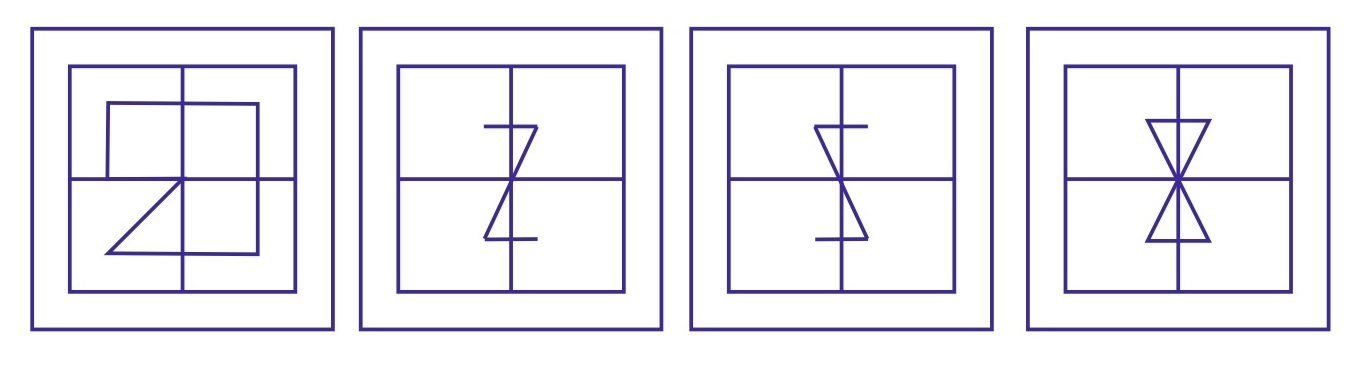(A)                                     (B)                            (C)                        (D)

Ans .   D

You can comment or ask me anything in comment sections related to embedded figure test questions and answers. To more practice, embedded figure test non-verbal reasoning questions and answers visit the next page.